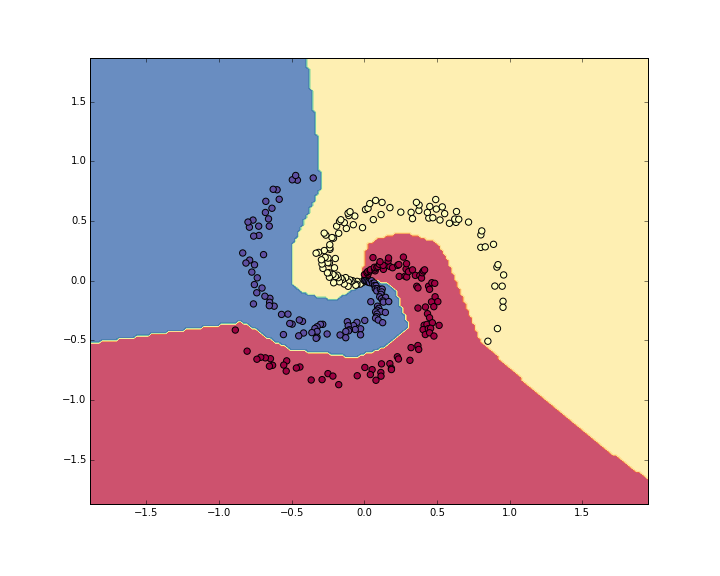# 神经网络简单实现

Posted by jjx on March 9, 2017

N = 100 # 每个类中的样本点
D = 2 # 维度
K = 3 # 类别个数
X = np.zeros((N*K,D)) # 样本input
y = np.zeros(N*K, dtype='uint8') # 类别标签
for j in xrange(K):
ix = range(N*j,N*(j+1))
t = np.linspace(j*4,(j+1)*4,N) + np.random.randn(N)*0.2 # theta
X[ix] = np.c_[r*np.sin(t), r*np.cos(t)]
y[ix] = j
# 可视化一下我们的样本点
plt.scatter(X[:, 0], X[:, 1], c=y, s=40, cmap=plt.cm.Spectral)
plt.xlim([-1,1])
plt.ylim([-1,1])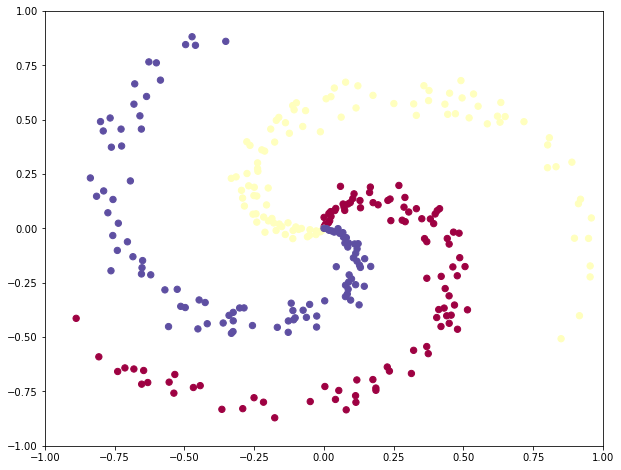#### 使用Softmax线性分类器

#随机初始化参数
import numpy as np
#D=2表示维度，K=3表示类别数
W = 0.01 * np.random.randn(D,K)
b = np.zeros((1,K))


#使用得分函数计算得分
scores = np.dot(X, W) + b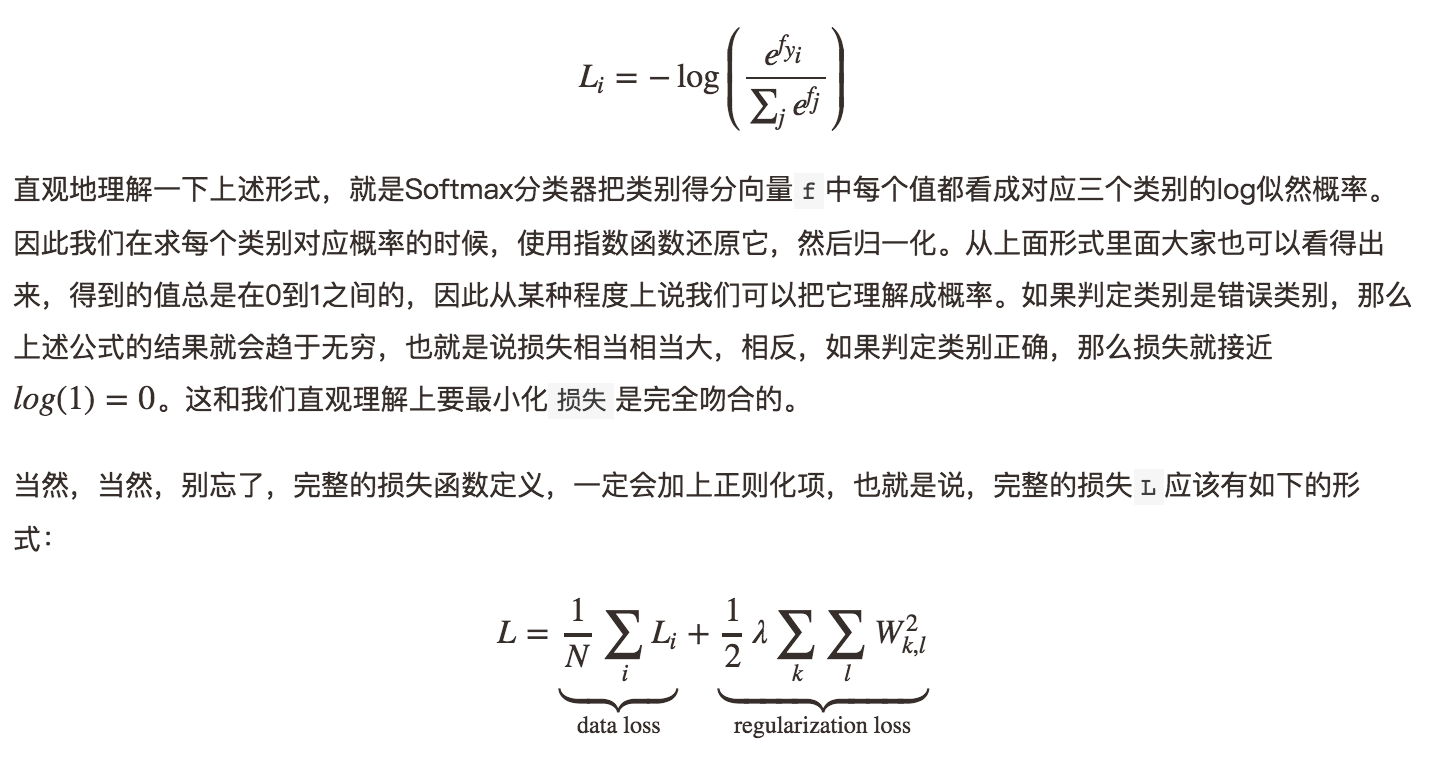# 用指数函数还原
exp_scores = np.exp(scores)
# 归一化
probs = exp_scores / np.sum(exp_scores, axis=1, keepdims=True)


#计算log概率和互熵损失
corect_logprobs = -np.log(probs[range(num_examples),y])
data_loss = np.sum(corect_logprobs)/num_examples
#加上正则化项
reg_loss = 0.5*reg*np.sum(W*W)
loss = data_loss + reg_loss


dscores = probs
dscores[range(num_examples),y] -= 1
dscores /= num_examples


dW = np.dot(X.T, dscores)
db = np.sum(dscores, axis=0, keepdims=True)
#得记着正则化梯度哈
dW += reg*W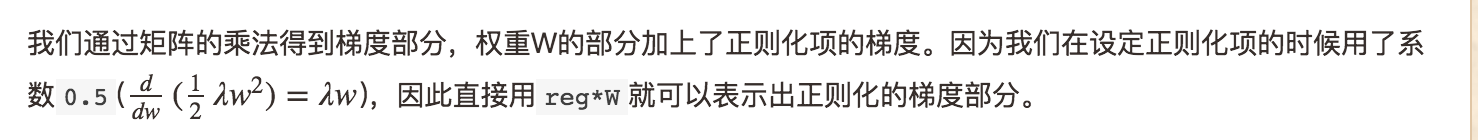#参数迭代更新
W += -step_size * dW
b += -step_size * db


#代码部分组一起，训练线性分类器

#随机初始化参数
W = 0.01 * np.random.randn(D,K)
b = np.zeros((1,K))

#需要自己敲定的步长和正则化系数
step_size = 1e-0
reg = 1e-3 #正则化系数

#梯度下降迭代循环
num_examples = X.shape
for i in xrange(200):

# 计算类别得分, 结果矩阵为[N x K]
scores = np.dot(X, W) + b

# 计算类别概率
exp_scores = np.exp(scores)
probs = exp_scores / np.sum(exp_scores, axis=1, keepdims=True) # [N x K]

# 计算损失loss(包括互熵损失和正则化部分)
corect_logprobs = -np.log(probs[range(num_examples),y])
data_loss = np.sum(corect_logprobs)/num_examples
reg_loss = 0.5*reg*np.sum(W*W)
loss = data_loss + reg_loss
if i % 10 == 0:
print "iteration %d: loss %f" % (i, loss)

# 计算得分上的梯度
dscores = probs
dscores[range(num_examples),y] -= 1
dscores /= num_examples

# 计算和回传梯度
dW = np.dot(X.T, dscores)
db = np.sum(dscores, axis=0, keepdims=True)

dW += reg*W # 正则化梯度

#参数更新
W += -step_size * dW
b += -step_size * db


iteration 0: loss 1.096956
iteration 10: loss 0.917265
iteration 20: loss 0.851503
iteration 30: loss 0.822336
iteration 40: loss 0.807586
iteration 50: loss 0.799448
iteration 60: loss 0.794681
iteration 70: loss 0.791764
iteration 80: loss 0.789920
iteration 90: loss 0.788726
iteration 100: loss 0.787938
iteration 110: loss 0.787409
iteration 120: loss 0.787049
iteration 130: loss 0.786803
iteration 140: loss 0.786633
iteration 150: loss 0.786514
iteration 160: loss 0.786431
iteration 170: loss 0.786373
iteration 180: loss 0.786331
iteration 190: loss 0.786302


190次循环之后，结果大致收敛了。我们评估一下准确度：

#评估准确度
scores = np.dot(X, W) + b
predicted_class = np.argmax(scores, axis=1)
print 'training accuracy: %.2f' % (np.mean(predicted_class == y))


# plot the resulting classifier
h = 0.02
x_min, x_max = X[:, 0].min() - 1, X[:, 0].max() + 1
y_min, y_max = X[:, 1].min() - 1, X[:, 1].max() + 1
xx, yy = np.meshgrid(np.arange(x_min, x_max, h),
np.arange(y_min, y_max, h))
Z = np.dot(np.c_[xx.ravel(), yy.ravel()], W) + b
Z = np.argmax(Z, axis=1)
Z = Z.reshape(xx.shape)
fig = plt.figure()
plt.contourf(xx, yy, Z, cmap=plt.cm.Spectral, alpha=0.8)
plt.scatter(X[:, 0], X[:, 1], c=y, s=40, cmap=plt.cm.Spectral)
plt.xlim(xx.min(), xx.max())
plt.ylim(yy.min(), yy.max())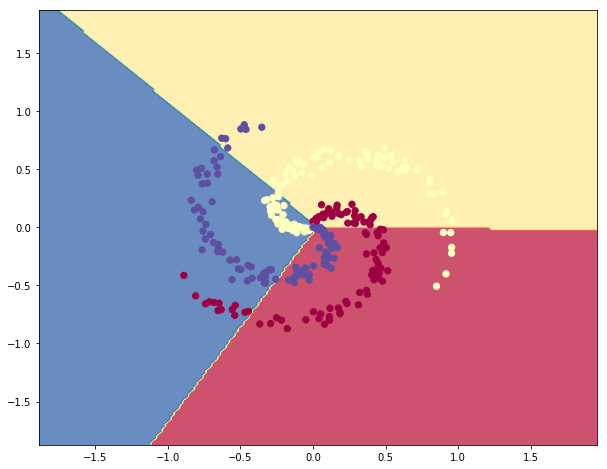#### 使用神经网络分类

# 初始化参数
h = 100 # 隐层大小(神经元个数)
W = 0.01 * np.random.randn(D,h)
b = np.zeros((1,h))
W2 = 0.01 * np.random.randn(h,K)
b2 = np.zeros((1,K))


#2层神经网络的前向计算
hidden_layer = np.maximum(0, np.dot(X, W) + b) # 用的 ReLU单元
scores = np.dot(hidden_layer, W2) + b2


# 梯度回传与反向传播
# 对W2和b2的第一次计算
dW2 = np.dot(hidden_layer.T, dscores)
db2 = np.sum(dscores, axis=0, keepdims=True)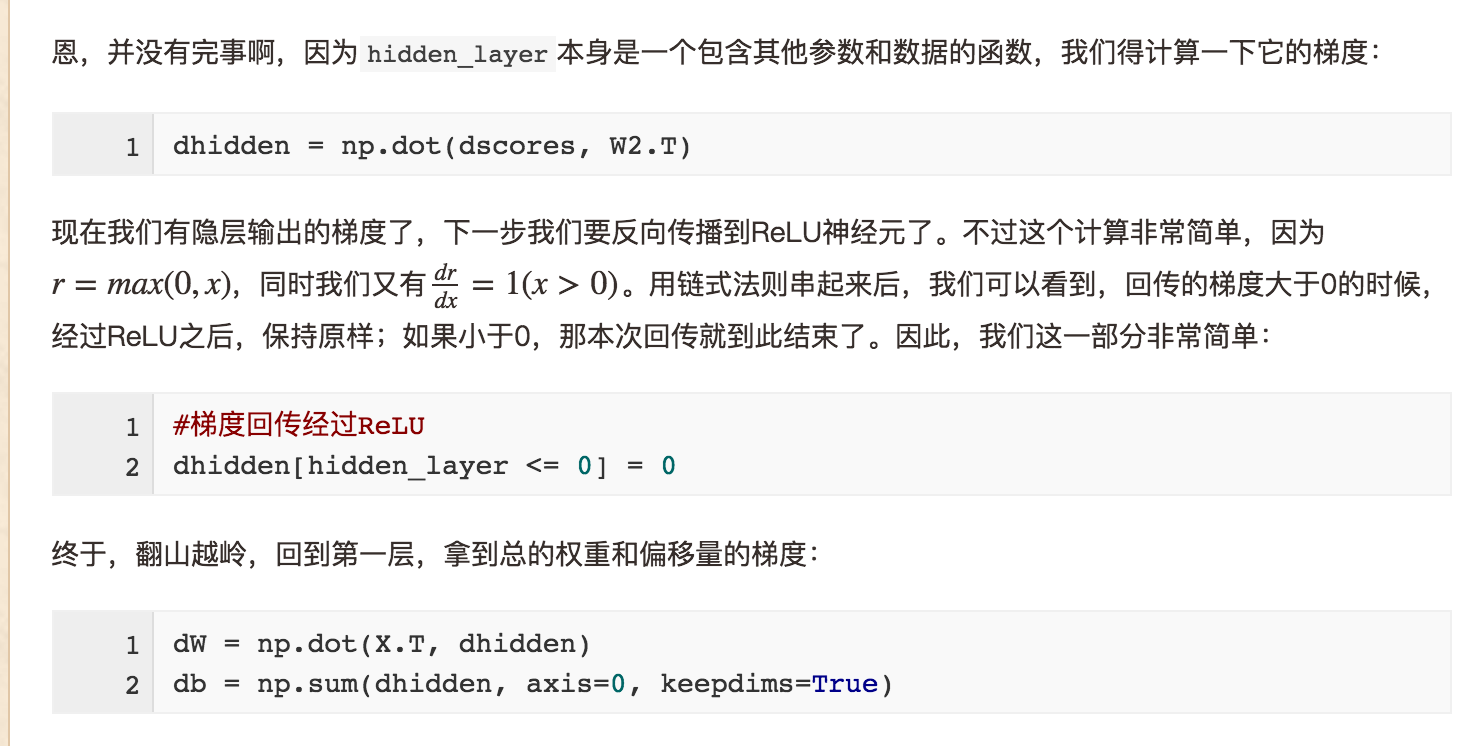# 随机初始化参数
h = 100 # 隐层大小
W = 0.01 * np.random.randn(D,h)
b = np.zeros((1,h))
W2 = 0.01 * np.random.randn(h,K)
b2 = np.zeros((1,K))

# 手动敲定的几个参数
step_size = 1e-0
reg = 1e-3 # 正则化参数

# 梯度迭代与循环
num_examples = X.shape
for i in xrange(10000):

hidden_layer = np.maximum(0, np.dot(X, W) + b) #使用的ReLU神经元
scores = np.dot(hidden_layer, W2) + b2

# 计算类别概率
exp_scores = np.exp(scores)
probs = exp_scores / np.sum(exp_scores, axis=1, keepdims=True) # [N x K]

# 计算互熵损失与正则化项
corect_logprobs = -np.log(probs[range(num_examples),y])
data_loss = np.sum(corect_logprobs)/num_examples
reg_loss = 0.5*reg*np.sum(W*W) + 0.5*reg*np.sum(W2*W2)
loss = data_loss + reg_loss
if i % 1000 == 0:
print "iteration %d: loss %f" % (i, loss)

# 计算梯度
dscores = probs
dscores[range(num_examples),y] -= 1
dscores /= num_examples

# 梯度回传
dW2 = np.dot(hidden_layer.T, dscores)
db2 = np.sum(dscores, axis=0, keepdims=True)

dhidden = np.dot(dscores, W2.T)

dhidden[hidden_layer <= 0] = 0
# 拿到最后W,b上的梯度
dW = np.dot(X.T, dhidden)
db = np.sum(dhidden, axis=0, keepdims=True)

# 加上正则化梯度部分
dW2 += reg * W2
dW += reg * W

# 参数迭代与更新
W += -step_size * dW
b += -step_size * db
W2 += -step_size * dW2
b2 += -step_size * db2


iteration 0: loss 1.098744
iteration 1000: loss 0.294946
iteration 2000: loss 0.259301
iteration 3000: loss 0.248310
iteration 4000: loss 0.246170
iteration 5000: loss 0.245649
iteration 6000: loss 0.245491
iteration 7000: loss 0.245400
iteration 8000: loss 0.245335
iteration 9000: loss 0.245292


#计算分类准确度
hidden_layer = np.maximum(0, np.dot(X, W) + b)
scores = np.dot(hidden_layer, W2) + b2
predicted_class = np.argmax(scores, axis=1)
print 'training accuracy: %.2f' % (np.mean(predicted_class == y))